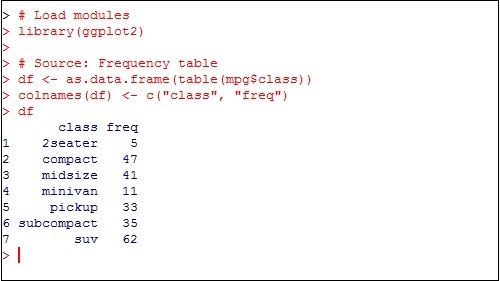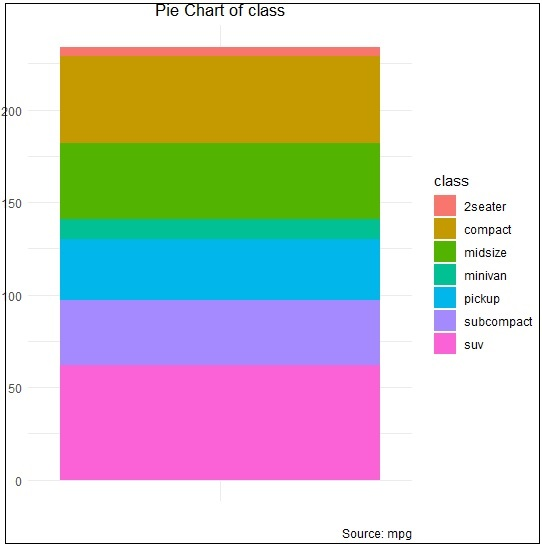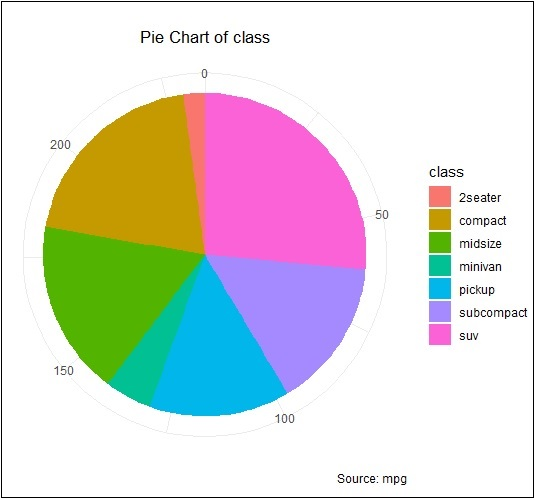# ggplot2 - Pie Charts

A pie chart is considered as a circular statistical graph, which is divided into slices to illustrate numerical proportion. In the mentioned pie chart, the arc length of each slice is proportional to the quantity it represents. The arc length represents the angle of pie chart. The total degrees of pie chart are 360 degrees. The semicircle or semi pie chart comprises of 180 degrees.

## Creating Pie Charts

Load the package in the mentioned workspace as shown below −

```> # Load modules
> library(ggplot2)
>
> # Source: Frequency table
> df <- as.data.frame(table(mpg\$class))
> colnames(df) <- c("class", "freq")
```The sample chart can be created using the following command −

```> pie <- ggplot(df, aes(x = "", y=freq, fill = factor(class))) +
+ geom_bar(width = 1, stat = "identity") +
+ theme(axis.line = element_blank(),
+    plot.title = element_text(hjust=0.5)) +
+    labs(fill="class",
+       x=NULL,
+       y=NULL,
+       title="Pie Chart of class",
+       caption="Source: mpg")
> pie
```

If you observe the output, the diagram is not created in circular manner as mentioned below −## Creating co-ordinates

Let us execute the following command to create required pie chart as follows −

```> pie + coord_polar(theta = "y", start=0)
```